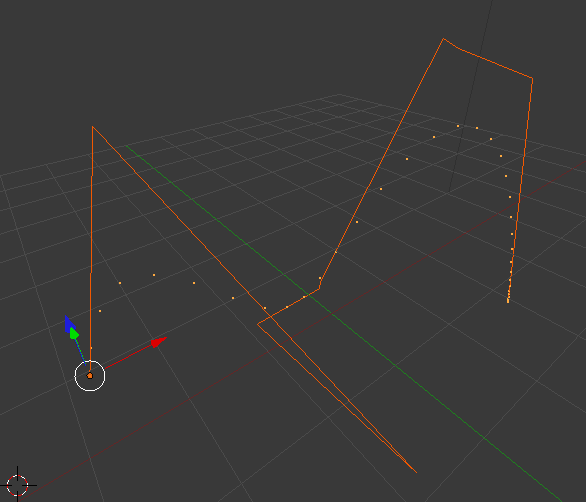Your browser (Internet Explorer 6) is out of date. It has known security flaws and may not display all features of this and other websites. Learn how to update your browser.
X
The de Casteljau Algorithm

# The de Casteljau Algorithm

This is a follow up to Blender and Blossoms.

The de Casteljau Algorithm is a recursive way to calculate the Point on a Bézier curve given its control polygon $P = \{ \vec{b_i} \}$ consisting of the Points $b_i$. It is defined as follows in the Book:

Given the points $\vec{b}_0 ... \vec{b}_n$, $t \in \mathbb{R}$ and a function $f(t): \mathbb{R} \to \mathbb{R}$. Set

\begin{eqnarray*}\vec{b}_i^0 & := & \vec{b_i} \\ \vec{b}_i^r & := & (1-f(t)) \vec{b}_i^{r-1} + f(t)\,\vec{b}_{i+1}^{r-1}(t)\end{eqnarray*}

Then $\vec{b}_0^n$ is the point with the parameter $t$ on the corresponding Beziér curve.

So this is essentially a recursive definition: Starting from $n$ points, we always combine two of them and remain with $n-1$ points. We continue this scheme until only one point is left. This is the point on the curve for the value $t$.

# Implementation

The de Casteljau Algorithm is short and beautifully implemented:

import numpy as np

class DeCasteljau:
def __init__(self, *pts, func = lambda t: t):
self._b = np.asarray(pts, dtype=np.float64)
self._func = func

def __call__(self, t):
b, n = self._b, len(self._b)
for r in range(1,n):
for i in range(n-r):
b[i] = (1-self._func(t))*b[i] + self._func(t)*b[i+1]

return b


And this is how it looks.The orange curve is the defining polygon and the dots are the corresponding beziér curve evaluated for 100 values of $t \in [0,1]$ and $f$ being the identity.

You can find the complete code in the GitHub repository under chap04.